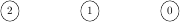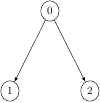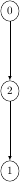Queries for Posets: search statistic / browse statistics / browse maps from / browse maps to

# 1. Definition & Example

• A (finite) poset (or partially ordered set) is a finite set $P$ together with a partial order $\leq$ satisfying

• $\leq$ is reflexive: $x \leq x$ for all $x \in P$,

• $\leq$ is transitive, $x \leq y \leq z$ implies $x \leq z$ for all $x,y,z \in P$,

• $\leq$ is antisymmetric, $x \leq y \Rightarrow y \not\leq x$ for $x,y \in P$ such that $x \neq y$,

see [Bru10] and [Sta10].

• One often writes $a < b$ for $a \leq b$ and $a \neq b$.

• A cover relation $a \prec b$ is a pair of elements $a < b$ such that there exists no $c \in P$ for which $a < c < b$ [Wei14].

 the 5 Posets of size 3([],3) ([(1,2)],3) ([(0,1),(0,2)],3) ([(0,2),(2,1)],3) ([(0,2),(1,2)],3)
• Posets are graphically represented by their Hasse diagram which is the directed graph of cover relations.

• Two posets $(P,\leq_P)$ and $(P',\leq_{P'})$ are isomorphic if there exists a bijection $\pi: P\ \tilde\longrightarrow\ P'$ such that $x \leq_P y$ if and only if $\pi(x) \leq_{P'} \pi(y)$ for all $x,y \in P$.

• This project considers unlabelled posets. This is, two posets are considered to be equal if they are isomorphic.

• For the number of unlabelled posets see OEIS:A000112.

# 2. Additional information

## 2.1. Notations

• $x,y \in P$ are called comparable if $x \leq y$ or $y \leq x$. A poset is called linear, linearly ordered, or totally ordered if any two elements are comparable.

• $x \in P$ is minimal if there is no $y \in X$ such that $y \leq x$,

• $x \in P$ is maximal if there is no $y \in X$ such that $x \leq y$.

• A subset $X$ of $P$ is called chain if it is pairwise comparable, and antichain if it is pairwise not comparable. A maximal chain is containment-maximal chain.

• An order ideal or down-closed set is a subset $X$ of $P$ such that $x \leq y \in X$ implies $x \in X$. There is a one-to-one correspondence between order ideals and antichains.

## 2.2. Extensions of Posets

• Let $P$ be a set with two partial orders $\leq_1$ and $\leq_2$. Then $\leq_2$ is an extension of $\leq_1$ if $x \leq_1 y$ implies $x \leq_2 y$.

• Linear extensions of posets play a particularly important role, see [Sta10].

[Bru10]   R. Brualdi, Partial orders and equivalence relations, Combinatorics Fifth Edition (2010).

[Sta10]   R. Stanley, Enumerative Combinatorics Vol 1, Second edition, Cambridge Studies in Advanced Mathematics (2011).

[Wei14]   E. Weisstein, Cover relation, Math World (2014).

# 5. Technical information for database usage

• A poset is uniquely represented as a tuple (E,n) where E is the sorted list of cover relations and n is the number of elements. For this representation, we consider a canonical labelling of a poset. This is, a labelling of the elements by $\{0,1,\ldots,n-1\}$ such that any two posets are isomorphic if and only if their canonical labellings coincide.

• Posets are graded by the number of elements.
• The database contains all posets of size at most 7.